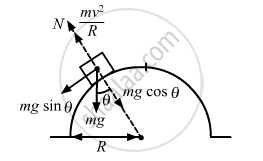Share

# A Motorcycle is Going on an Overbridge of Radius R. the Driver Maintains a Constant Speed. as the Motorcycle is Ascending on the Overbridge - Physics

ConceptCircular Motion

#### Question

A motorcycle is going on an overbridge of radius R. The driver maintains a constant speed. As the motorcycle is ascending on the overbridge, the normal force on it

•  increases

• decreases

•  remains the same

• fluctuates.

#### SolutionThe normal force on the motorcycle , $\text{N = mg}\cos\theta - \frac{\text{mv}^2}{R}$

As the motorcycle is ascending on the overbridge, θ decreases (from $\frac{\pi}{2}$ to 0).

So, normal force increases with decrease in θ.

Is there an error in this question or solution?

#### APPEARS IN

Solution A Motorcycle is Going on an Overbridge of Radius R. the Driver Maintains a Constant Speed. as the Motorcycle is Ascending on the Overbridge Concept: Circular Motion.
S